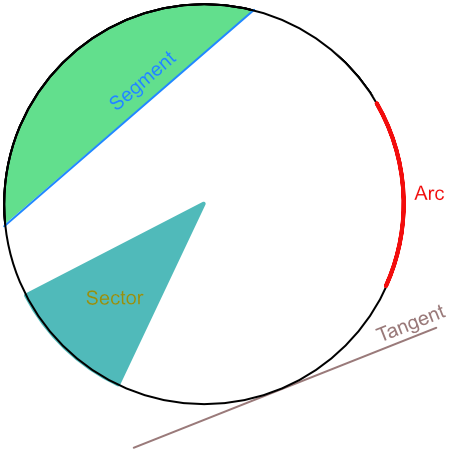Parts of Circles

# Parts of Circles

GCSE(F), GCSE(H),

A tangent is a line drawn outside a circle that only touches the circumference at one point. The angle between a target and a radius drawn to that same point is a right angle.

A sector is an area of the circle drawn between two radii. It forms a slice of a circle. A sector is normally measured in degrees.

An arc is part of a circumference.

The area of a sector is bound by two radii and an arc.

A segment is an area that is bounded by a chord and by the circumference of the circle between the points of the chord. Where a segment is exactly half a circle, it is called a semicircle.## Examples

1. A sector has an angle of 60º. What fraction of the complete circle does the sector represent?

Answer: frac(1)(6)

A circle has 360º. The arc is 60º, therefore represents frac(60)(360) = frac(1)(6) of the circle.

2. A tangent is drawn to a circle. How many degrees are there between the tangent to the circle, and a radius that touches that same point on the circle?